The field of data mining has made significant advances in recent years. Because of its ability to solve complex problems, data mining has been applied in diverse fields related to engineering, biological science, social media, medicine, and business intelligence. The primary objective for most of the applications is to characterize patterns in a complex stream of data. These patterns are then coupled with knowledge discovery and decision making. In the Internet age, information gathering and dynamic analysis of spatiotemporal data are key to innovation and developing better products and processes. When datasets are large and complex, it becomes difficult to process and analyze patterns using traditional statistical methods. Big data are data collected in volumes so large, and forms so complex and unstructured, that they cannot be handled using standard database management systems, such as DBMS and RDBMS. The emerging challenges associated with big data include dealing not only with increased volume, but also the wide variety and complexity of the data streams that need to be extracted, transformed, analyzed, stored, and visualized. Big data analysis uses inferential statistics to draw conclusions related to dependencies, behaviors, and predictions from large sets of data with low information density that are subject to random variations. Such systems are expected to model knowledge discovery in a format that produces reasonable answers when applied across a wide range of situations. The characteristics of big data are as follows:

• Volume: A great quantity of data is generated. Detecting relevance and value within this large volume is challenging.

• Variety: The range of data types and sources is wide.

• Velocity: The speed of data generation is fast. Reacting in a timely manner can be demanding.

• Variability: Data flows can be highly inconsistent and difficult to manage, owing to seasonal and event-driven peaks.

• Complexity: The data need to be linked, connected, and correlated to infer nonlinear relationships and causal effects.

Modern technological advancements have enabled the industry to make inroads into big data and big data analytics. Affordable open source software infrastructure, faster processors, cheaper storage, virtualization, high throughput connectivity, and development of unstructured data management tools, in conjunction with cloud computing, have opened the door to high-quality information retrieval and faster analytics, enabling businesses to reduce costs and time required to develop newer products with customized offerings.

Big data and powerful analytics can be integrated to deliver valuable services, such as these:

• Failure root cause detection: The cost of unplanned shutdowns resulting from unexpected failures can run into billions of dollars. Root cause analysis (RCA) identifies the factors determinative of the location, magnitude, timing, and nature of past failures and learns to associate actions, conditions, and behaviors that can prevent the recurrence of such failures. RCA transforms a reactive approach to failure mitigation into a proactive approach of solving problems before they occur and avoids unnecessary escalation.

• Dynamic coupon system: A dynamic coupon system allows discount coupons to be delivered in a very selective manner, corresponding to factors that maximize the strategic benefits to the product or service provider. Factors that regulate the delivery of the coupon to selected recipients are modeled on existing locality, assessed interest in a specific product, historical spending patterns, dynamic pricing, chronological visits to shopping locations, product browsing patterns, and redemption of past coupons. Each of these factors is weighted and reanalyzed as a function of competitive pressures, transforming behaviors, seasonal effects, external factors, and dynamics of product maturity. A coupon is delivered in real time, according to the recipient’s profile, context, and location. The speed, precision, and accuracy of coupon delivery to large numbers of mobile recipients are important considerations.

• Shopping behavior analysis: A manufacturer of a product is particularly interested in the understanding the heat-map patterns of its competitors’ products on the store floor. For example, a manufacturer of large-screen TVs would want to ascertain buyers’ interest in features offered by other TV manufacturers. This can only be analyzed by evaluating potential buyers’ movements and time spent in proximity to the competitors’ products on the floor. Such reports can be delivered to the manufacturer on an individual basis, in real time, or collectively, at regular intervals. The reports may prompt manufacturers to deliver dynamic coupons to influence potential buyers who are still in the decision-making stage as well as help the manufacturer improve, remove, retain, or augment features, as gauged by buyers’ interest in the competitors’ products.

• Detecting fraudulent behavior: Various types of fraud related to insurance, health care, credit cards, and identity theft cost consumers and businesses billions of dollars. Big data and smart analytics have paved the way for developing real-time solutions for identifying fraud and preventing it before it occurs. Smart analytics generate models that validate the patterns related to spending behavior, geolocation, peak activity, and insurance claims. If a pattern cannot be validated, a corrective, preventive, or punitive action is initiated. The accuracy, precision, and velocity of such actions are critical to the success of isolating the fraudulent behavior. For instance, each transaction may evaluate up to 500 attributes, using one or more models in real time.

## Knowledge Discovery

Knowledge extraction gathers information from structured and unstructured sources to construct a knowledge database for identifying meaningful and useful patterns from underlying large and semantically fuzzy datasets. Fuzzy datasets are sets whose elements have a degree of membership. Degree of membership is defined by a membership function that is valued between 0 and 1.

The extracted knowledge is reused, in conjunction with source data, to produce an enumeration of patterns that are added back to the knowledge base. The process of knowledge discovery involves programmatic exploration of large volumes of data for patterns that can be enumerated as knowledge. The knowledge acquired is presented as models to which specific queries can be made, as necessary. Knowledge discovery joins the concepts of computer science and machine learning (such as databases and algorithms) with those of statistics to solve user-oriented queries and issues. Knowledge can be described in different forms, such as classes of actors, attribute association models, and dependencies. Knowledge discovery in big data uses core machine algorithms that are designed for classification, clustering, dimensionality reduction, and collaborative filtering as well as scalable distributed systems. This chapter discusses the classes of machine learning algorithms that are useful when the dataset to be processed is very large for a single machine.

### Classification

Classification is central to developing predictive analytics capable of replicating human decision making. Classification algorithms work well for problems with well-defined boundaries in which inputs follow a specific set of attributes and in which the output is categorical. Generally, the classification process develops an archive of experiences entailing evaluation of new inputs by matching them with previously observed patterns. If a pattern can be matched, the input is associated with the predefined predictive behavioral pattern. If a pattern cannot be matched, it is quarantined for further evaluation to determine if it is an undiscovered valid pattern or an unusual pattern. Machine-based classification algorithms follow supervised-learning techniques, in which algorithms learn through examples (also called training sets) of accurate decision making, using carefully prepared inputs. The two main steps involved in classification are synthesizing a model, using a learning algorithm, and employing the model to categorize new data.

### Clustering

Clustering is a process of knowledge discovery that groups items from a given collection, based on similar attributes (or characteristics). Members of the same cluster share similar characteristics, relative to those belonging to different clusters. Generally, clustering involves an iterative algorithm of trial and error that operates on an assumption of similarity (or dissimilarity) and that stops when a termination criterion is satisfied. The challenge is to find a function that measures the degree of similarity between two items (or data points) as a numerical value. The parameters for clustering—such as the clustering algorithm, the distance function, the density threshold, and the number of clusters—depend on the applications and the individual dataset.

### Dimensionality Reduction

Dimensionality reduction is the process of reducing random variables through feature selection and feature extraction. Dimensionality reduction allows shorter training times and enhanced generalization and reduces overfitting. Feature selection is the process of synthesizing a subset of the original variables for model construction by eliminating redundant or irrelevant features. Feature extraction, in contrast, is the process of transforming the high-dimensional space to a space of fewer dimensions by combining attributes.

### Collaborative Filtering

Collaborative filtering (CF) is the process of filtering for information or patterns, using collaborative methods between multiple data sources. CF explores an area of interest by gathering preferences from many users with similar interests and making recommendations based on those preferences. CF algorithms are expected to make satisfactory recommendations in a short period of time, despite very sparse data, increasing numbers of users and items, synonymy, data noise, and privacy issues.

Machine learning performs predictive analysis, based on established properties learned from the training data (models). Machine learning assists in exploring useful knowledge or previously unknown knowledge by matching new information with historical information that exists in the form of patterns. These patterns are used to filter out new information or patterns. Once this new information is validated against a set of linked behavioral patterns, it is integrated into the existing knowledge database. The new information may also correct existing models by acting as additional training data. The following sections look at various machine learning algorithms employed in knowledge discovery, in relation to clustering, classification, dimensionality reduction, and collaborative filtering.

## Machine Learning: Classification Algorithms

### Logistic Regression

Logistic regression is a probabilistic statistical classification model that predicts the probability of the occurrence of an event. Logistic regression models the relationship between a categorical dependent variable X and a dichotomous categorical outcome or feature Y. The logistic function can be expressed as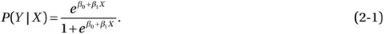The logistic function may be rewritten and transformed as the inverse of the logistic function—called logit or log-odds—which is the key to generating the coefficients of the logistic regression,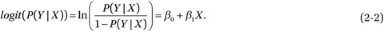As depicted in Figure 2-1, the logistic function can receive a range of input values (β 0 +β 1 X) between negative infinity and positive infinity, and the output (P(Y|X) is constrained to values between 0 and 1.

The logit transform of P(Y|X) provides a dynamic range for linear regression and can be converted back into odds. The logistic regression method fits a regression curve, using the regression coefficients β 0 and β 1, as shown in Equation 2-1, where the output response is a binary (dichotomous) variable, and X is numerical. Because the logistic function curve is nonlinear, the logit transform (see Equation 2-2) is used to perform linear regression, in which P(Y  |X) is the probability of success (Y) for a given value of X. Using the generalized linear model, an estimated logistic regression equation can be formulated as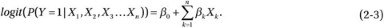The coefficients β 0 and β k (k = 1, 2, ..., n) are estimated, using maximum likelihood estimation (MLE) to model the probability that the dependent variable Y will take on a value of 1 for given values of X k (k = 1, 2, ..., n).

Logistic regression is widely used in areas in which the outcome is presented in a binary format. For example, to predict blood cholesterol based on body mass index (BMI), you would use linear regression, because the outcome is continuous. If you needed to predict the odds of being diabetic based on BMI, you would use logistic regression, because the outcome is binary.

### Random Forest

Random forest (Breiman 2001) is an ensemble learning approach for classification, in which “weak learners” collaborate to form “strong learners,” using a large collection of decorrelated decision trees (the random forest). Instead of developing a solution based on the output of a single deep tree, however, random forest aggregates the output from a number of shallow trees, forming an additional layer to bagging. Bagging constructs n predictors, using independent successive trees, by bootstrapping samples of the dataset. The n predictors are combined to solve a classification or estimation problem through averaging. Although individual classifiers are weak learners, all the classifiers combined form a strong learner. Whereas single decision trees experience high variance and high bias, random forest averages multiple decision trees to improve estimation performance. A decision tree, in ensemble terms, represents a weak classifier. The term forest denotes the use of a number of decision trees to make a classification decision.

The random forest algorithm can be summarized as follows:

1. 1.

To construct B trees, select n bootstrap samples from the original dataset.

2. 2.

For each bootstrap sample, grow a classification or regression tree.

3. 3.

At each node of the tree:

m predictor variables (or subset of features) are selected at random from all the predictor variables (random subspace).

– The predictor variable that provides the best split performs the binary split on that node.

– The next node randomly selects another set of m variables from all predictor variables and performs the preceding step.

4. 4.

Given a new dataset to be classified, take the majority vote of all the B subtrees.

By averaging across the ensemble of trees, you can reduce the variance of the final estimation. Random forest offers good accuracy and runs efficiently on large datasets. It is an effective method for estimating missing data and maintains accuracy, even if a large portion of the data is missing. Additionally, random forest can estimate the relative importance of a variable for classification.

### Hidden Markov Model

A hidden Markov model (HMM) is a doubly stochastic process, in which the system being modeled is a Markov process with unobserved (hidden) states. Although the underlying stochastic process is hidden and not directly observable, it can be seen through another set of stochastic processes that produces the sequence of observed symbols. In traditional Markov models, states are visible to an observer, and state transitions are parameterized, using transition probabilities. Each state has a probability distribution over output emissions (observed variables). HMM-based approaches correlate the system observations and state transitions to predict the most probable state sequence. The states of the HMM can only be inferred from the observed emissions—hence, the use of the term hidden. The sequence of output emissions generated by an HMM is used to estimate the sequence of states. HMMs are generative models, in which the joint distribution of observations and hidden states is modeled. To define a hidden Markov model, the following attributes have to be specified (see Figure 2-2):

• Set of states: {S 1,S 2...,S n}

• Sequence of states: Q=q 1,q 2,...,q t

• Markov chain property: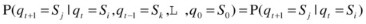• Set of observations: O={o 1,o 2,o 3,...,o M}

• Transition probability matrix: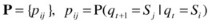• Emission probability matrix: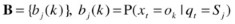• Initial probability matrix: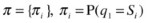• HMM: M = (A,B,π)

The three fundamental problems addressed by HMMs can be summarized as follows:

• Model evaluation: Evaluate the likelihood of a sequence of observations for a given HMM (M=(A,B,π)).

• Path decoding: Evaluate the optimal sequence of model states (Q) (hidden states) for a given sequence of observations and HMM model M=(A,B,π).

• Model training: Determine the set of model parameters that best accounts for the observed signal.

HMMs are especially known for their application in temporal pattern recognition, such as speech, handwriting, gesture recognition, part-of-speech tagging, musical score following, partial discharges, and bioinformatics. For further details on the HMM, see Chapter 5.

### Multilayer Perceptron

A multilayer perceptron (MLP) is a feedforward network of simple neurons that maps sets of input data onto a set of outputs. An MLP comprises multiple layers of nodes fully connected by directed graph, in which each node (except input nodes) is a neuron with a nonlinear activation function.

The fundamental component of an MLP is the neuron. In an MLP a pair of neurons is connected in two adjacent layers, using weighted edges. As illustrated in Figure 2-3, an MLP comprises at least three layers of neurons, including one input layer, one or more hidden layers, and one output layer. The number of input neurons depends on the dimensions of the input features; the number of output neurons is determined by the number of classes. The number of hidden layers and the number of neurons in each hidden layer depend on the type of problem being solved. Fewer neurons result in inefficient learning; a larger number of neurons results in inefficient generalization. An MLP uses a supervised-learning technique called backpropagation for training the network. In its simple instantiation the perceptron computes an output y by processing a linear combination of weighted real-valued inputs through a nonlinear activation function,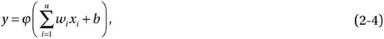where w represents the weights vector, x is the input vector, b is the bias, and ϕ is the activation function. Generally, MLP systems choose the logistic sigmoid function 1/(1+e x) or the hyperbolic tangent tanh(x) as the activation functions. These functions offer statistical convenience, because they are linear near the origin and saturate quickly when moved away from the origin.

The MLP learning process adjusts the weights of the hidden layer, such that the output error is reduced. Starting with the random weights, MLP feeds forward the input pattern signals through the network and backpropagates the error signal, starting at the output. The backpropagating error signal is made up of of the difference between actual (O n(t)) and desired (T n) values. Error function may be summarized as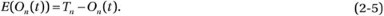The goal of the learning process is to minimize the error function. To find the minimum value of the error function, differentiate it, with respect to the weight matrix. The learning algorithm comprises the following steps:

1. 1.

Initialize random weights within the interval [1, –1].

2. 2.

Send an input pattern to the network.

3. 3.

Calculate the output of the network.

4. 4.

For each node n in the output layer:

1. a.

Calculate the error on output node n: E(O n(t))=T nO n(t).

2. b.

Add E(O n(t)) to all the weights that connect to node n.

5. 5.

Repeat step 2.

To influence the convergence rate and thereby reduce the step sizes at which weights undergo an adaptive change, a learning parameter η (< 1) is used. The i-th weight connected to j-th output can be updated by the following rule: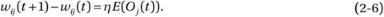Equation 2-6 represents an iterative weight adaptation, in which a fraction of output error at iteration (t + 1) is added to the existing weight from iteration t.

MLPs are commonly used for supervised-learning pattern recognition processes. There is renewed interest in MLP backpropagation networks, owing to the successes of deep learning. Deep learning is an approach for effectively training an MLP, using multiple hidden layers. With modern advancements in silicon technology, deep learning is being developed to unlock the enormous big data analytics potential in areas in which highly varying functions can be represented by deep architecture.

## Machine Learning: Clustering Algorithms

### k-Means Clustering

k-means clustering is an unsupervised-learning algorithm of vector quantization that partitions n observations into k clusters. The algorithm defines k centroids, which act as prototypes for their respective clusters. Each object is assigned to a cluster with the nearest centroid when measured with a specific distance metric. The step of assigning objects to clusters is complete when all the objects have been applied to one of the k clusters. The process is repeated by recalculating centroids, based on previous S={S 1,S 1,...,S k} allocations, and reassigning objects to the nearest new centroids. The process continues until there is no movement of centroids of any k cluster. Generally, a k-means clustering algorithm classifies objects according to their features into k groups (or clusters) by minimizing the sum of squares of the distances between the object data and the cluster centroid.

For a given set of d-dimensional observations vectors (x 1,x 2,...,x n), k-means clustering partitions n observations into k(≤n) cluster sets so as to minimize the sum of squares,where μ i is the mean of the points in S i.

The k-means clustering algorithm is easy to implement on large datasets. It has found many uses in areas such as market segmentation, computer vision, profiling applications and workloads, optical character recognition, and speech synthesis. The algorithm is often used as the preprocessing step for other algorithms in order to find the initial configuration.

### Fuzzy k-Means (Fuzzy c-Means)

Fuzzy k-means (also called fuzzy c-means [FCM]) (Dunn 1973; Bezdek 1981) is an extension of the k-means algorithm that synthesizes soft clusters, in which an object can belong to more than one cluster with a certain probability. This algorithm provides increased flexibility in assigning data objects to clusters and allowing the data objects to maintain partial membership in multiple neighboring clusters. FCM uses the fuzzification parameter m in range [1, n], which determines the degree of fuzziness in the clusters. Whereas m=1 signifies crisp clustering, m>1 suggests a higher degree of fuzziness among data objects in decision space. The FCM algorithm is based on minimization of the objective functionwhere x is the d-dimensional data object, c j is the d-dimensional centroid of the cluster j (see Equation 2-10), and w k(x) is the degree of membership of x in the cluster k dependent on the fuzzification parameter m, which controls the weighting accorded the closest centroid: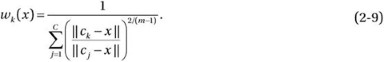With FCM the d-dimensional centroid of a kth cluster (c k) is the mean of all points, weighted by their degree of membership to that cluster: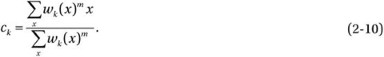The c-means clustering algorithm synthesizes cluster centers and the degree to which data objects are assigned to them. This does not translate into hard membership functions. FCM is used in image processing for clustering objects in an image.

### Streaming k-Means

Streaming k-means is a two-step algorithm, consisting of a streaming step and a ball k-means step. A streaming step traverses the data objects of size n in one pass and generates an optimal number of centroids—which amounts to klog(n) clusters, where k is expected number of clusters. The attributes of these clusters are passed on to the ball k-means step, which reduces the number of clusters to k.

#### Streaming Step

A streaming-step algorithm steps through the data objects one at a time and makes a decision to either add the data object to an existing cluster or create a new one. If the distance between the centroid of the cluster and a data point is smaller than the distance cutoff threshold, the algorithm adds the data to an existing cluster or creates a new cluster with a probability of d/(distancecutoff). If the distance exceeds the cutoff, the algorithm creates a new cluster with a new centroid. As more data objects are processed, the centroids of the existing clusters may change their position. This process continues to add new clusters until the number of existing clusters reaches a cluster cutoff limit. The number of clusters can be reduced by increasing the distance cutoff threshold. This step is mainly used for dimensionality reduction. The output of this step is a reduced dataset in the form of multiple clusters that are proxies for a large amount of the original data.

#### Ball K-Means Step

A ball k-means algorithm consumes the output of a streaming step (X = set of centroids > k) and performs multiple independent runs to synthesize k clusters by selecting the best solution. Each run selects k centroids, using a seeding mechanism, and runs the ball k-means algorithm iteratively to refine the solution.

The seeding process may invoke the k-means++ algorithm for optimal spreading of k clusters. The k-means++ seeding algorithm is summarized as follows:

1. 1.

Choose center c 1 uniformly at random from X.

2. 2.

Select a new center c i by choosing xϵX with probability, P(x), and add it to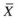,where D(x) is the distance between x and the nearest center that has already been chosen.

1. 3.

Repeat step 2 until k centers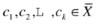are selected.

2. 4.

Randomly pick two centers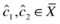with probability proportional to norm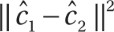.

3. 5.

For each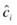, create a ball of radius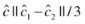around it.

4. 6.

Recompute the new centroids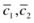by using the elements ofcontained within the ball.

This algorithm is particularly useful in applications with a large number of data objects. The algorithm reduces the dimensionality of the original dataset by employing the streaming operation and replacing that data with a reduced proxy data composed of k·log(n) centroids of the original data. The reduced data act as input to the ball k-means algorithm, which synthesizes and refines k centroids for their respective clusters.

## Machine Learning: Dimensionality Reduction

Machine learning works through a large number of features to train most regression or classification problems. This compounds the complexity, raises the computational requirement, and increases the time needed to converge to a solution. A useful approach for mitigating these problems is to reduce the dimensional space of the original features by synthesizing a lower-dimensional space. In this new, lower-dimensional space the most important features are retained, hidden correlations between features are exposed, and unimportant features are discarded. One of the simplest, most straightforward, and least supervised feature-reduction approaches involves variants of matrix decomposition: singular value decomposition, eigen decomposition, and nonnegative matrix factorization. The following sections consider some of the methods commonly used in statistical dimensionality reduction.

### Singular Value Decomposition

Singular value decomposition (SVD) performs matrix analysis to synthesize low-dimensional representation of a high-dimensional matrix. SVD assists in eliminating less important parts of matrix representation, leading to approximate representation with the desired number of dimensions. This helps in creating a smaller representation of a matrix that closely resembles the original. SVD is useful in dimensionality reduction, owing to the following characteristics:

• SVD transforms correlated variables into a set of uncorrelated ones that exposes corresponding relationships between the data items.

• SVD identifies dimensions along which data points exhibit the most variation.

Once you identify the points with distinct variations, you can approximate original data points with fewer dimensions. You can define thresholds below which variations can be ignored, thereby leading to a highly reduced dataset without degradation of the information related to inherent relationships and interests within data points.

If M is an m × n matrix, then you can break it down into the product of three matrices U, ∑, and V T with the following characteristics:

• U is a column-orthogonal matrix. The columns of U are orthonormal eigenvectors of MM   T.

• V   T is a transpose of orthogonal matrix V. The columns of V are orthonormal eigenvectors of M   T M.

• ∑ is a diagonal matrix, where all elements except diagonal are 0. ∑ contains square roots of eigenvalues from U or V, in descending order.

In its exact form, M can be rewritten asIn the process of dimensionality reduction, you synthesize U and V, such that they contain elements accounted for in the original data, in descending order of variation. You may delete elements representing dimensions that do not exhibit meaningful variation. This can be done by setting the smallest eigenvalue to 0. Equation 2-11 can be rewritten in its best rank-l approximate form as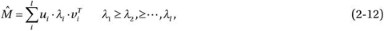where u i and v i are the ith columns of U and V, respectively, and λ i is the ith element of the diagonal matrix ∑.

### Principal Component Analysis

When you have a swarm of points in space, the coordinates and axes you use to represent such points are arbitrary. The points have certain variances, relative to the direction of axes chosen, indicating the spread around the mean value in that direction. In a two-dimensional system the model is constrained by the perpendicularity of the second axis to the first axis. But, in three-dimensional cases and higher, you can position the nth axis perpendicular to the plane constructed by any two axes. The model is constrained by the position of the first axis, which is positioned in the direction with the highest variance. This results in a new feature space that compresses the swarm of points into the axes of high variance. You may select the axes with higher variances and eliminate the axes with lower variances. Figure 2-4 illustrates the new feature space, reduced from a dataset with 160 featuresto 59 components (axes). Each component is associated with a certain percentage of variance, relative to other components. The first component has the highest variance, followed by second component, and so on.

Principal component analysis (PCA) is a widely used analytic technique that identifies patterns to reduce the dimensions of the dataset without significant loss of information. The goal of PCA is to project a high-dimensional feature space into a smaller subset to decrease computational cost. PCA computes new features, called principal components (PCs), which are uncorrelated linear combinations of the original features projected in the direction of greater variability. The key is to map the set of features into a matrix M and synthesize the eigenvalues and eigenvectors for MM   T or M   T M. Eigenvectors facilitate simpler solutions to problems that can be modeled using linear transformations along axes by stretching, compressing, or flipping. Eigenvalues provide a factor (length and magnitude of eigenvectors) whereby such transformation occurs. Eigenvectors with larger eigenvalues are selected in the new feature space because they enclose more information than eigenvectors with lower eigenvalues for a data distribution. The first PC has the greatest possible variance (i.e., the largest eigenvalues) compared with the next PC (uncorrelated, relative to the first PC), which is computed under the constraint of being orthogonal to the first component. Essentially, the ith PC is the linear combination of the maximum variance that is uncorrelated with all previous PCs.

PCA comprises the following steps:

1. 1.

Compute the d-dimensional mean of the original dataset.

2. 2.

Compute the covariance matrix of the features.

3. 3.

Compute the eigenvectors and eigenvalues of the covariance matrix.

4. 4.

Sort the eigenvectors by decreasing eigenvalue.

5. 5.

Choose k eigenvectors with the largest eigenvalues.

Eigenvector values represent the contribution of each variable to the PC axis. PCs are oriented in the direction of maximum variance in m-dimensional points.

PCA is one of the most widely used multivariate methods for uncovering new, informative, uncorrelated features; it reduces dimensionality by rejecting low-variance features and is useful in reducing the computational requirements for classification and regression analysis.

### Lanczos Algorithm

The Lanczos algorithm is a low-cost eigen-decomposition technique identical to truncated SVD, except that it does not explicitly compute singular values/vectors of the matrix. The Lanczos algorithm uses a small number of Lanczos vectors that are eigenvectors of M T M or MM T, where M is a symmetrical n × n matrix.

Lanczos starts by seeding an arbitrary nonzero vector x 0 with cardinality equal to the number of columns of matrix M. The mth (m<<n) step of the algorithm transforms the matrix M into a tridiagonal matrix T mm. The iterative process can be summarized as follows:

#### Initialize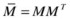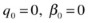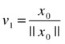#### Algorithm

FOR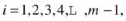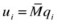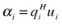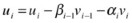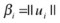IF β i=0, then STOP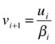END

After m iterations are completed, you get α i and β i, which are the diagonal and subdiagonal entries, respectively, of the symmetrical tridiagonal matrix T mm. The resulting tridiagonal matrix is orthogonally similar to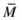: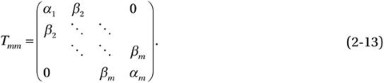The symmetrical tridiagonal matrix represents the projections of given matrices onto a subspace spanned by corresponding sets of Lanczos vectors V m. The eigenvalues of these matrices are the eigenvalues of the mapped subspace of the original matrix. Lanczos iterations by themselves do not directly produce eigenvalues or eigenvectors; rather, they produce a tridiagonal matrix (see Equation 2-13) whose eigenvalues and eigenvectors are computed by another method (such as the QR algorithm) to produce Ritz values and vectors. For the eigenvalues, you may compute the k smallest or largest eigenvalues of T mm if the number of Lanczos iterations is large compared with k. The Lanczos vectors v i so generated then construct the transformation matrix,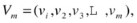which can be used to generate the Ritz eigenvectors (V m·u m), the approximate eigenvectors to the original matrix.

## Machine Learning: Collaborative Filtering

Collaborative filtering (CF is used by recommender systems, whose goal is to forecast the user’s interest in a given item, based on collective user experience (collaboration). The main objective is to match people with similar interests to generate personalized recommendations. Let’s say, for instance, that there are M items and N users. This gives us an M × N user–item matrix X, where x m,n represents n th user recommendations for item m. The following sections discuss some of the CF systems used in recommender systems.

### User-Based Collaborative Filtering

User-based CF forecasts the user’s interest in an item, based on collective ratings from similar user profiles. The user–item matrix can be written as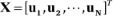The first step in user-based CF is to evaluate the similarity between users and arrange them according to their nearest neighbor. For example, to evaluate the similarity between two users, you may use a cosine similarity matrix u n ,u a: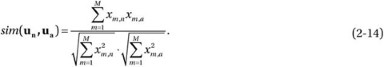Finally, the predicted rating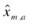of test item m by test user a is computed as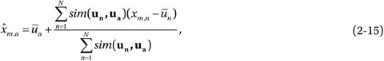where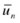and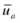denote the average rating made by users n and a, respectively. As seen from Equations 2-14 and 2-15, processing CF is a compute-intensive job function and may require large resource pools and faster computing machines. Therefore, it is recommended that you leverage a Hadoop platform for better performance and scalability.

### Item-Based Collaborative Filtering

Item-based CF computes the similarity between items and selects the best match. The idea is to isolate users that have reviewed both items and then compute the similarity between them. The user–item matrix is represented as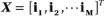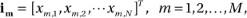where i m corresponds to an item’s ratings by all users m, which results in item-based recommendation algorithms.

The first step in item-based CF is to evaluate the similarity between items and arrange them according to their nearest neighbor. For instance, you may use the cosine similarity matrix to evaluate the similarity between two items i m ,i b. To remove the difference in rating scale between users when computing the similarity, the cosine similarity is adjusted by subtracting the user’s average rating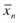(Sarwar 2001) from each co-rated pair: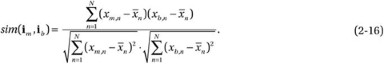Finally, the predicted ratingof test item m by test user a is computed as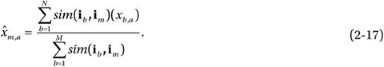The rating of an item by a user can be estimated by averaging the ratings of similar items evaluated by the same user.

### Alternating Least Squares with Weighted-λ-Regularization

The alternating-least-squares with weighted-λ-regularization (ALS-WR)algorithm factors the user–item matrix into the user–factor matrix and the item–factor matrix. This algorithm strives to uncover the latent factors that rationalize the observed user–item ratings and searches for optimal factor weights to minimize the least squares between predicted and actual ratings (Zhou 2008).

If you have multiple users and items, you will need to learn the feature vectors that represent each item and each user in the feature space. The objective is to uncover features that associate each user u with a user–factor vector, and each item i with an item–factor vector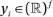. Ratings are described by the inner dot product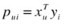of the user–factor vector and the item–factor vector. The idea is to perform matrix factorization, such that users and items can be mapped into common latent factors, whereby they can be directly compared. Because the rating matrix is sparse and not fully defined, the factorization has to be done using known ratings only. The quality of the solution is measured not only with respect to the observed data, but also with respect to a generalization of the unobserved data. You have to find a set of user and item feature vectors that minimizes the following cost function: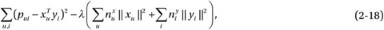where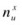andrepresent the number of ratings of user u and item i, respectively. The regularization term λ(...) avoids overfitting the training data. The parameter λ depends on the data and is tuned by cross-validation in the dataset for better generalization. Because the search space is very large (multiple users and items), it prevents application of traditional direct optimization techniques, such as stochastic gradient descent.

The cost function assumes a quadratic form when either the user–factor or the item–factor is fixed, which allows computation of a global minimum. This in turn allows ALS optimization, in which user–factors and item–factors are alternately recomputed by fixing each other. This algorithm is designed for large-scale CF for large datasets.

## Machine Learning: Similarity Matrix

A similarity matrix scores the similarity between data points. Similarity matrices are strongly related to their counterparts: distance matrices and substitution matrices. The following sections look at some of the commonly used similarity calculation methods.

### Pearson Correlation Coefficient

Pearson correlation measures the linear dependence between two variables. The Pearson correlation coefficient is the covariance of the two variables (X and Y) divided by the product of their standard deviations: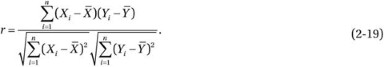The Pearson correlation coefficient ranges from −1 to 1. A value of 1 validates a perfect linear relationship between X and Y, in which the data variability of X tracks that of Y. A value of −1 indicates a reverse relationship between X and Y, such that the data variability of Y is opposite to that of X. A value of 0 suggests lack of linear correlation between the variables X and Y.

Although the Pearson coefficient reflects the strength of the linear relationship, it is highly sensitive to extreme values and outliers. The low relationship strength may be misleading if two variables have a strong curvilinear relationship instead of a strong linear relationship. The coefficient may also be misleading if X and Y have not been analyzed in terms of their full ranges.

### Spearman Rank Correlation Coefficient

The Spearman correlation coefficient performs statistical analysis of the strength of a monotonic relationship between the paired variables X and Y. Spearman correlation calculates Pearson correlation for the ranked values of the paired variables. Ranking (from low to high) is obtained by assigning a rank of 1 to the lowest value, 2 to the next lowest, and so on, such that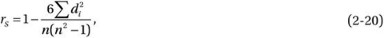where n is the sample size, and d is the distance between the statistical ranks of the variable pairs given by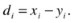The sign of the Spearman correlation coefficient signifies the direction of the association between the dependent and independent variables. The coefficient is positive if the dependent variable Y increases (or decreases) in the same direction as the independent variable X. The coefficient is negative if the dependent variable Y increases (or decreases) in the reverse direction, relative to the independent variable X. A Spearman correlation of 0 signifies that the variable Y has no inclination to either increase or decrease, relative to X. Spearman correlation increases in magnitude as X and Y move closer to being perfect monotone functions. Spearman correlation can only be computed if the data are not truncated. Although less sensitive to extreme values, it relies only on rank instead of observation.

### Euclidean Distance

The Euclidean distance is the square root of the sum of squared differences between the vector elements of the two variables: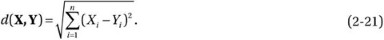A Euclidean distance is valid if both variables are measured on the same scale. You can transform the distance in Equation 2-21 to an inverse form (see Equation 2-22), such that it returns a value of 1 if X and Y (X – Y = 0) are similar and trend to 0 if the similarity decreases: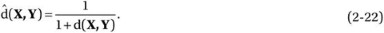You can verify that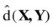calculates to the value of 1 if the distance d(X,Y) = 0 (indicating similarity), anddecreases to 0 if d(X,Y) increases (indicating dissimilarity).

### Jaccard Similarity Coefficient

The Jaccard similarity coefficient gauges similarity between finite sample sets X and Y by measuring overlapping between them. Sets X and Y do not have to be of same size. Mathematically, the coefficient can be defined as the ratio of the intersection to the union of the sample sets (X, Y):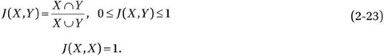The Jaccard distance measures the dissimilarity between sample sets and is obtained by subtracting the Jaccard coefficient from 1:The Jaccard coefficient is commonly used in measuring keyword similarities, document similarities, news article classification, natural language processing (NLP), and so on.

## Summary

The solution to a complex problem relies on intelligent use of machine learning techniques. The precision, speed, and accuracy of the solution can be improved by employing techniques that not only reduce the dimensionality of the features, but also train the models specific to a unique behavior. Distinct behavioral attributes can be clustered into phases by using one of the clustering techniques, such as k-means. Reduced data points corresponding to each cluster label are separated and trained to solve a regression or classification problem. In a normal posttraining operation, once phases are identified, the trained model associated with that phase is employed to forecast (or estimate) the output of the feedback loop.

Figure 2-5 summarizes a process control system capable of sensing a large number of sensors in order to control an environmental process (e.g., cooling in the datacenter). The first step is to reduce the dimensionality of the data. The new data are fed into clustering methods, which discover a group’s items from a given collection, based on similar attributes and distinctive properties. Data corresponding to each cluster label are segregated and trained individually for classification. Phase identification allows the application of a model function, which associates with the identified phase. The output of the phase-specific model triggers the process control functions, which act on the environment and change the sensor outputs. Additionally, this procedure lets us actively predict the current phase duration and the upcoming phase and accordingly forecast the output for proactive control.